# Python 3 Tutorial

Python is a high-level programming language and is widely being used among the developers’ community. Python was mainly developed for emphasis on code readability, and its syntax allows programmers to express concepts in fewer lines of code. Python is a programming language that lets developers work quickly and integrate systems more efficiently.
This Python 3 tutorial provides learners (either beginner or experienced developer) with the topics from Python basics to advanced topics with examples.Key features

Python has many reasons for being popular and in demand. A few of the reasons are mentioned below.

• Emphasis on code readability, shorter codes, ease of writing.
• Programmers can express logical concepts in fewer lines of code in comparison to languages such as C++ or Java.
• Python supports multiple programming paradigms, like object-oriented, imperative and functional programming or procedural.
• It provides extensive support libraries(Django for web development, Pandas for data analytics etc)
• Dynamically typed language(Data type is based on value assigned)
• Philosophy is “Simplicity is the best”.

# Getting started with Python

Python is a lot easier to code and learn. Python programs can be written on any plain text editor like notepad, notepad++, or anything of that sort. One can also use an online IDE for writing Python codes or can even install one on their system to make it more feasible to write these codes because IDEs provide a lot of features like intuitive code editor, debugger, compiler, etc.
To begin with, writing Python Codes and performing various intriguing and useful operations, one must have Python installed on their System. This can be done by following the step by step instructions provided below:

What if Python already exists? Let’s check
Windows don’t come with Python preinstalled, it needs to be installed explicitly. But unlike windows, most of the Linux OS have Python pre-installed, also macOS comes with Python pre-installed.
To check if your device is pre-installed with Python or not, just go to Command Line(For Windows, search for cmd in the Run dialog( + R), for Linux open the terminal using `Ctrl+Alt+T`, for macOS use `control+Option+Shift+T`.

Now run the following command:
For Python3

```python3 --version
```

If Python is already installed, it will generate a message with the Python version available.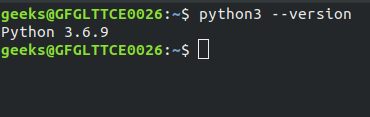Before starting with the installation process, you need to download it. For that all versions of Python for Windows, Linux, and MacOS are available on python.org.

For almost every Linux system, the following commands would work definitely.

```\$ sudo add-apt-repository ppa:deadsnakes/ppa
\$ sudo apt-get update
\$ sudo apt-get install python3.8
```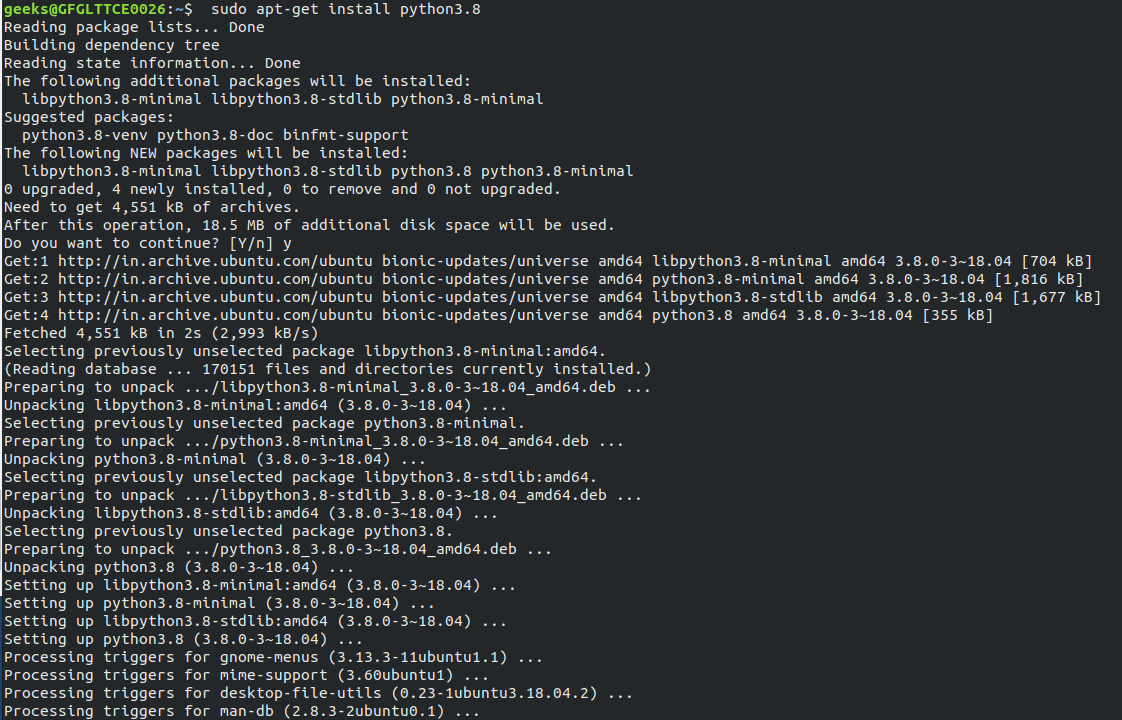To verify the installation enter following commands in your Terminal.

```python3
```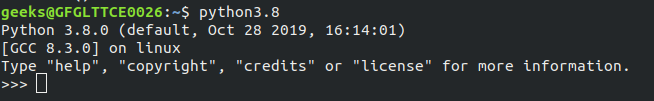### How to run a Python program

Let’s consider a simple Hello World Program.

```
# Python program to print
# Hello World

print("Hello World")

```

Generally, there are two ways to run a Python program.

• Using IDEs: You can use various IDEs(Pycharm, Jupyter Notebook, etc.) which can be used to run Python programs.
• Using Command-Line: You can also use command line options to run a Python program. Below steps demonstrate how to run a Python program on Command line in Windows/Unix Operating System:

Windows

Open Commandline and then to compile the code type python HelloWorld.py. If your code has no error then it will execute properly and output will be displayed.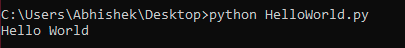Unix/Linux

Open Terminal of your Unix/Linux OS and then to compile the code type python HelloWorld.py. If your code has no error then it will execute properly and output will be displayed.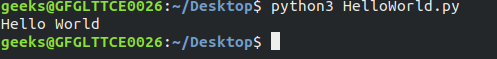# Fundamentals of Python

## Python Indentation

Python uses indentation to highlight the blocks of code. Whitespace is used for indentation in Python. All statements with the same distance to the right belong to the same block of code. If a block has to be more deeply nested, it is simply indented further to the right. You can understand it better by looking at the following lines of code.

```
# Python program showing
# indentation

site = 'gfg'

if site == 'gfg':
print('Logging on to geeksforgeeks...')
else:
print('retype the URL.')
print('All set !')
```

Output:

```Logging on to geeksforgeeks...
All set !
```

The lines `print(‘Logging on to geeksforgeeks…’)` and `print(‘retype the URL.’)` are two separate code blocks. The two blocks of code in our example if-statement are both indented four spaces. The final `print(‘All set!’)` is not indented, and so it does not belong to the else-block.

Comments are useful information that the developers provide to make the reader understand the source code. It explains the logic or a part of it used in the code. There are two types of comment in Python:

• Single line comments: Python single line comment starts with hashtag symbol with no white spaces.
```
# This is a comment
# Print “GeeksforGeeks !” to console
print("GeeksforGeeks")

```
• Multi-line string as comment: Python multi-line comment is a piece of text enclosed in a delimiter (“””) on each end of the comment.
```
"""
This would be a multiline comment in Python that
spans several lines and describes geeksforgeeks.
A Computer Science portal for geeks. It contains
well written, well thought
and well-explained computer science
and programming articles,
quizzes and more.
…
"""
print("GeeksForGeeks")
```

## Variables

Variables in Python are not “statically typed”. We do not need to declare variables before using them or declare their type. A variable is created the moment we first assign a value to it.

```
#!/usr/bin/python

# An integer assignment
age = 45

# A floating point
salary = 1456.8

# A string
name = "John"

print(age)
print(salary)
print(name)

```

Output:

```45
1456.8
John
```

## Operators

Operators are the main building block of any programming language. Operators allow the programmer to perform different kinds of operations on operands. These operators can be categorized based upon their different functionality:

• Arithmetic operators: Arithmetic operators are used to perform mathematical operations like addition, subtraction, multiplication and division.
```
# Examples of Arithmetic Operator
a = 9
b = 4

# Subtraction of numbers
sub = a - b
# Multiplication of number
mul = a * b
# Division(float) of number
div1 = a / b
# Division(floor) of number
div2 = a // b
# Modulo of both number
mod = a % b

# print results
print(sub)
print(mul)
print(div1)
print(div2)
print(mod)

```

Output:

```13
5
36
2.25
2
1
```
• Relational Operators: Relational operators compares the values. It either returns True or False according to the condition.
```
# Examples of Relational Operators
a = 13
b = 33

# a > b is False
print(a > b)

# a < b is True
print(a < b)

# a == b is False
print(a == b)

# a != b is True
print(a != b)

# a >= b is False
print(a >= b)

# a <= b is True
print(a <= b)

```

Output:

```False
True
False
True
False
True
```
• Logical Operators: Logical operators perform Logical AND, Logical OR and Logical NOT operations.
```
# Examples of Logical Operator
a = True
b = False

# Print a and b is False
print(a and b)

# Print a or b is True
print(a or b)

# Print not a is False
print(not a)

```

Output:

```False
True
False
```
• Bitwise operators: Bitwise operator acts on bits and performs bit by bit operation.
```
# Examples of Bitwise operators
a = 10
b = 4

# Print bitwise AND operation
print(a & b)

# Print bitwise OR operation
print(a | b)

# Print bitwise NOT operation
print(~a)

# print bitwise XOR operation
print(a ^ b)

# print bitwise right shift operation
print(a >> 2)

# print bitwise left shift operation
print(a << 2)
```

Output:

```0
14
-11
14
2
40
```
• Assignment operators: Assignment operators are used to assign values to the variables.
• Special operators: Special operators are of two types-
• Identity operator that contains `is` and `is not`.
• Membership operator that contains `in` and `not in`.
```
# Examples of Identity and
# Membership operator

a1 = 'GeeksforGeeks'
b1 = 'GeeksforGeeks'

# Identity operator
print(a1 is not b1)
print(a1 is b1)

# Membership operator
print("G" in a1)
print("N" not in b1)

```

Output:

```False
True
True
True
```

# Basics of Input/Output

## Taking input from user –

Python provides us with two inbuilt functions to read the input from the keyboard.

• raw_input(): This function works in older version (like Python 2.x). This function takes exactly what is typed from the keyboard, convert it to string and then return it to the variable in which we want to store. For example:
```
# Python program showing
# a use of raw_input()

g = raw_input("Enter your name : ")
print g

```
• input(): This function first takes the input from the user and then evaluates the expression, which means Python automatically identifies whether the user entered a string or a number or list. For example:
```
# Python program showing
# a use of input()

val = input("Enter your value: ")
print(val)

```

## Printing output to console –

The simplest way to produce output is using the `print()` function where you can pass zero or more expressions separated by commas. This function converts the expressions you pass into a string before writing to the screen.

```
# Python 3.x program showing
# how to print data on
# a screen

# One object is passed
print("GeeksForGeeks")

x = 5
# Two objects are passed
print("x =", x)

# code for disabling the softspace feature
print('G', 'F', 'G', sep ='')

# using end argument
print("Python", end = '@')
print("GeeksforGeeks")

```

Output:

```GeeksForGeeks
x = 5
GFG
Python@GeeksforGeeks
```

# Data Types

Data types are the classification or categorization of data items. It represents the kind of value that tells what operations can be performed on a particular data. Since everything is an object in Python programming, data types are actually classes and variables are instance (object) of these classes.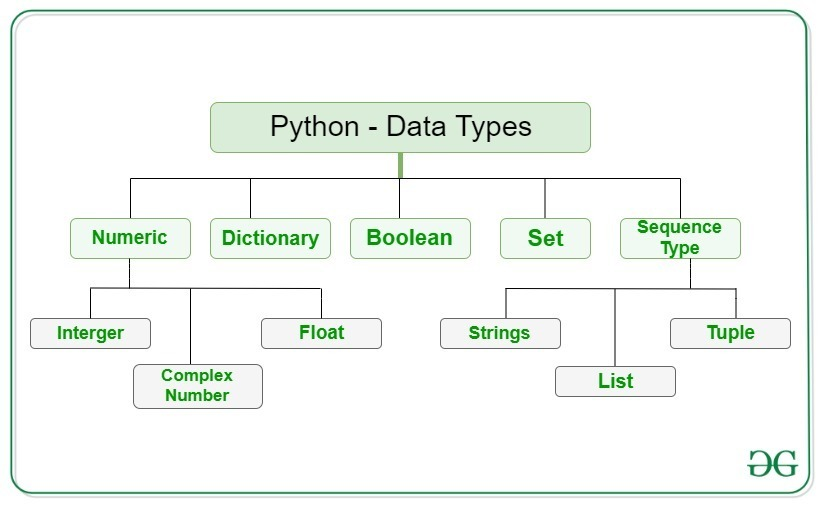## Numeric

In Python, numeric data type represent the data which has numeric value. Numeric value can be interger, floating number or even complex numbers. These values are defined as `int`, `float` and `complex` class in Python.

```
# Python program to
# demonstrate numeric value

print("Type of a: ", type(5))

print("\nType of b: ", type(5.0))

c = 2 + 4j
print("\nType of c: ", type(c))

```

Output:

```Type of a:  <class 'int'>

Type of b:  <class 'float'>

Type of c:  <class 'complex'>
```

## Sequence Type

In Python, a sequence is the ordered collection of similar or different data types. Sequences allow storing multiple values in an organized and efficient fashion. There are several sequence types in Python –

• String
• List
• Tuple

1) String: A string is a collection of one or more characters put in a single quote, double-quote or triple quote. In python there is no character data type, a character is a string of length one. It is represented by `str` class. Strings in Python can be created using single quotes or double quotes or even triple quotes.

```
# Python Program for
# Creation of String

# String with single quotes
print('Welcome to the Geeks World')

# String with double quotes
print("I'm a Geek")

# String with triple quotes
print('''I'm a Geek and I live in a world of "Geeks"''')

```

Output:

```Welcome to the Geeks World
I'm a Geek
I'm a Geek and I live in a world of "Geeks"
```

Accessing elements of string –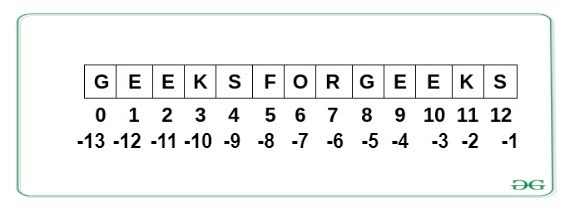```
# Python Program to Access
# characters of String

String1 = "GeeksForGeeks"

# Printing First character
print(String1)

# Printing Last character
print(String1[-1])

```

Output:

```G
s
```

Deleting/Updating from a String –

In Python, Updation or deletion of characters from a String is not allowed because Strings are immutable. Only new strings can be reassigned to the same name.

```
# Python Program to Update / delete
# character of a String

String1 = "Hello, I'm a Geek"

# Updating a character
String1 = 'p'

# Deleting a character
del String1

```

Output:

```Traceback (most recent call last):
File “/home/360bb1830c83a918fc78aa8979195653.py”, line 6, in
String1 = ‘p’
TypeError: ‘str’ object does not support item assignment

Traceback (most recent call last):
File “/home/499e96a61e19944e7e45b7a6e1276742.py”, line 8, in
del String1
TypeError: ‘str’ object doesn’t support item deletion
```

Refer to the below articles to know more about Strings:

2) List: Lists are just like the arrays, declared in other languages. A single list may contain DataTypes like Integers, Strings, as well as Objects. The elements in a list are indexed according to a definite sequence and the indexing of a list is done with 0 being the first index. It is represented by `list` class.

```
# Python program to demonstrate
# Creation of List

# Creating a List
List = []
print(List)

# Creating a list of strings
List = ['GeeksForGeeks', 'Geeks']
print(List)

# Creating a Multi-Dimensional List
List = [['Geeks', 'For'], ['Geeks']]
print(List)

```

Output:

```[]
['GeeksForGeeks', 'Geeks']
[['Geeks', 'For'], ['Geeks']]
```

Adding Elements to a List: Using `append()`, `insert()` and `extend()`

```
# Python program to demonstrate
# Addition of elements in a List

# Creating a List
List = []

# Using append()
List.append(1)
List.append(2)
print(List)

# Using insert()
List.insert(3, 12)
List.insert(0, 'Geeks')
print(List)

# Using extend()
List.extend([8, 'Geeks', 'Always'])
print(List)

```

Output:

```[1, 2]
['Geeks', 1, 2, 12]
['Geeks', 1, 2, 12, 8, 'Geeks', 'Always']
```

Accessing elements from the List –

Use the index operator `[ ]` to access an item in a list. In Python, negative sequence indexes represent positions from the end of the array. Instead of having to compute the offset as in `List[len(List)-3]`, it is enough to just write `List[-3]`.

```
# Python program to demonstrate
# accessing of element from list

List = [1, 2, 3, 4, 5, 6]

# accessing a element
print(List)
print(List)

# Negative indexing
# print the last element of list
print(List[-1])
# print the third last element of list
print(List[-3])

```

Output:

```1
3
6
4
```

Removing Elements from the List: Using `remove()` and `pop()`

```
# Python program to demonstrate
# Removal of elements in a List

# Creating a List
List = [1, 2, 3, 4, 5, 6,
7, 8, 9, 10, 11, 12]

# using Remove() method
List.remove(5)
List.remove(6)
print(List)

# using pop()
List.pop()
print(List)

```

Output:

```[1, 2, 3, 4, 7, 8, 9, 10, 11, 12]
[1, 2, 3, 4, 7, 8, 9, 10, 11]
```

Refer to the below articles to know more about List:

3) Tuple: Tuple is an ordered collection of Python objects much like a list. The important difference between a list and a tuple is that tuples are immutable. It is represented by `tuple` class. In Python, tuples are created by placing a sequence of values separated by ‘comma’ with or without the use of parentheses for grouping of the data sequence.

```
# Python program to demonstrate
# creation of Set

# Creating an empty tuple
Tuple1 = ()
print (Tuple1)

# Creating a tuple of strings
print(('Geeks', 'For'))

# Creating a Tuple of list
print(tuple([1, 2, 4, 5, 6]))

# Creating a nested Tuple
Tuple1 = (0, 1, 2, 3)
Tuple2 = ('python', 'geek')
Tuple3 = (Tuple1, Tuple2)
print(Tuple3)

```

Output:

```()
('Geeks', 'For')
(1, 2, 4, 5, 6)
((0, 1, 2, 3), ('python', 'geek'))
```

Accessing element of a tuple –

Use the index operator `[ ]` to access an item in a tuple.

```
# Python program to
# demonstrate accessing tuple

tuple1 = tuple([1, 2, 3, 4, 5])

# Accessing element using indexing
print(tuple1)

# Accessing element using Negative
# Indexing
print(tuple1[-1])

```

Output:

```1
5
```

Deleting/updating elements of tuple –

Items of a tuple cannot be deleted as tuples are immutable in Python. Only new tuples can be reassigned to the same name.

```
# Python program to
# demonstrate updation / deletion
# from a tuple

tuple1 = tuple([1, 2, 3, 4, 5])

# Updating an element
tuple1 = -1

# Deleting an element
del tuple1

```

Output:

```Traceback (most recent call last):
File "/home/084519a8889e9b0103b874bbbb93e1fb.py", line 11, in
tuple1 = -1
TypeError: 'tuple' object does not support item assignment

Traceback (most recent call last):
File "/home/ffb3f8be85dd393bde5d0483ff191343.py", line 12, in
del tuple1
TypeError: 'tuple' object doesn't support item deletion
```

Refer to the below articles to know more about tuples:

## Boolean

Booleans are data type with one of the two built-in values, `True` or `False`. It is denoted by the class bool.

```
# Python program to
# demonstrate boolean type

print(type(True))
print(1>2)
print('a'=='a')

```

Output:

```<class 'bool'>
False
True
```

## Set

In Python, Set is an unordered collection of data type that is iterable, mutable and has no duplicate elements. The order of elements in a set is undefined though it may consist of various elements. Sets can be created by using the built-in `set()` function with an iterable object or a sequence by placing the sequence inside curly braces `{}`, separated by ‘comma’.

```

# Python program to demonstrate
# Creation of Set in Python

# Creating a Set
set1 = set()

# Creating a Set of String
set1 = set("GeeksForGeeks")
print(set1)

# Creating a Set of List
set1 = set(["Geeks", "For", "Geeks"])
print(set1)

```

Output:

```{'o', 'r', 'k', 'G', 'e', 's', 'F'}
{'Geeks', 'For'}
```

Adding elements: Using `add()` and `update()`

```# Python program to demonstrate
# Addition of elements in a Set

set1 = set()

print(set1)

# Additio to the Set using Update()
set1.update([10, 11])
print(set1)
```

Output:

```{8, (6, 7)}
{8, 10, 11, (6, 7)}
```

Accessing a Set: One can loop through the set items using a `for` loop as set items cannot be accessed by referring to an index.

```
# Python program to demonstrate
# Accessing of elements in a set

# Creating a set
set1 = set(["Geeks", "For", "Geeks"])

# Accessing using for loop
for i in set1:
print(i, end =" ")

```

Output:

```Geeks For
```

Removing elements from a set: Using `remove()`, `discard()`, pop() and `clear()`

```
# Python program to demonstrate
# Deletion of elements in a Set

set1 = set([1, 2, 3, 4, 5, 6,
7, 8, 9, 10, 11, 12])

# using Remove() method
set1.remove(5)
set1.remove(6)
print(set1)

print(set1)

# Set using the pop() method
set1.pop()
print(set1)

# Set using clear() method
set1.clear()
print(set1)

```

Output:

```{1, 2, 3, 4, 7, 8, 9, 10, 11, 12}
{1, 2, 3, 4, 7, 10, 11, 12}
{2, 3, 4, 7, 10, 11, 12}
set()
```

Refer to the below articles to know more about Sets:

## Dictionary

Dictionary in Python is an unordered collection of data values, used to store data values like a map. Dictionary holds `key:value` pair. Each key-value pair in a Dictionary is separated by a colon `:`, whereas each key is separated by a ‘comma’. A Dictionary can be created by placing a sequence of elements within curly `{}` braces, separated by ‘comma’.

```
# Creating an empty Dictionary
Dict = {}
print(Dict)

# with Integer Keys
Dict = {1: 'Geeks', 2: 'For', 3: 'Geeks'}
print(Dict)

# with Mixed keys
Dict = {'Name': 'Geeks', 1: [1, 2, 3, 4]}
print(Dict)

```

Output:

```{}
{1: 'Geeks', 2: 'For', 3: 'Geeks'}
{1: [1, 2, 3, 4], 'Name': 'Geeks'}
```

Nested Dictionary: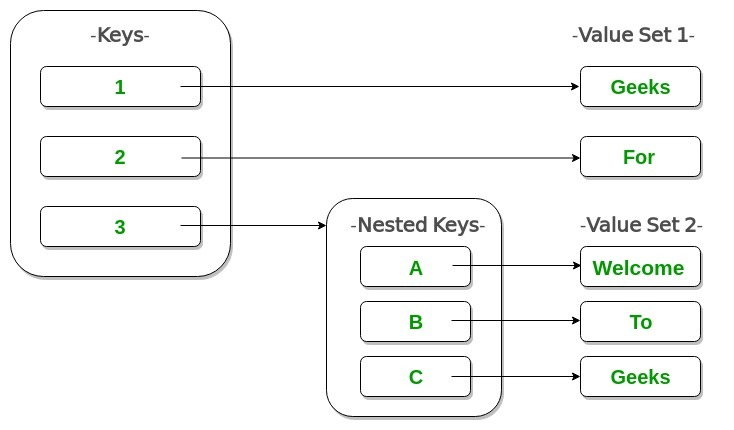```

# Creating a Nested Dictionary
# as shown in the below image
Dict = {1: 'Geeks', 2: 'For',
3:{'A' : 'Welcome', 'B' : 'To', 'C' : 'Geeks'}}

print(Dict)

```

Output:

```{1: 'Geeks', 2: 'For', 3: {'A': 'Welcome', 'B': 'To', 'C': 'Geeks'}}
```

Adding elements to a Dictionary: One value at a time can be added to a Dictionary by defining value along with the key e.g. `Dict[Key] = ‘Value’`.

```
# Creating an empty Dictionary
Dict = {}

# Adding elements one at a time
Dict = 'Geeks'
Dict = 'For'
Dict = 1
print(Dict)

# Updating existing Key's Value
Dict = 'Welcome'
print(Dict)

```

Output:

```{0: 'Geeks', 2: 'For', 3: 1}
{0: 'Geeks', 2: 'Welcome', 3: 1}
```

Accessing elements from a Dictionary: In order to access the items of a dictionary refer to its key name or use `get()` method.

```
# Python program to demonstrate
# accessing an element from a Dictionary

# Creating a Dictionary
Dict = {1: 'Geeks', 'name': 'For', 3: 'Geeks'}

# accessing a element using key
print(Dict['name'])

# accessing a element using get()
print(Dict.get(3))

```

Output:

```For
Geeks
```

Removing Elements from Dictionary: Using `pop()` and `popitem()`

```
# Initial Dictionary
Dict = { 5 : 'Welcome', 6 : 'To', 7 : 'Geeks',
'A' : {1 : 'Geeks', 2 : 'For', 3 : 'Geeks'},
}

# using pop()
Dict.pop(5)
print(Dict)

# using popitem()
Dict.popitem()
print(Dict)

```

Output:

```{'A': {1: 'Geeks', 2: 'For', 3: 'Geeks'}, 6: 'To', 7: 'Geeks'}
{6: 'To', 7: 'Geeks'}
```

Refer to the below articles to know more about dictionary:

# Decision Making

Decision Making in programming is similar to decision making in real life. A programming language uses control statements to control the flow of execution of the program based on certain conditions. These are used to cause the flow of execution to advance and branch based on changes to the state of a program.

Decision-making statements in Python

Example 1: To demonstrate `if` and `if-else`

```
# Python program to demonstrate
# decision making

a = 10
b = 15

# if to check even number
if a % 2 == 0:
print("Even Number")

# if-else to check even or odd
if b % 2 == 0:
print("Even Number")
else:
print("Odd Number")

```

Output:

```Even Number
Odd Number
```

Example 2: To demonstrate `nested-if` and `if-elif`

```
# Python program to demonstrate
# decision making

a = 10

# Nested if to check whether a
# number is divisible by both 2 and 5
if a % 2 == 0:
if a % 5 == 0:
print("Number is divisible by both 2 and 5")

# is-elif
if (a == 11):
print ("a is 11")
elif (a == 10):
print ("a is 10")
else:
print ("a is not present")

```

Output:

```Number is divisible by both 2 and 5
a is 10
```

# Control flow (Loops)

Loops in programming come into use when we need to repeatedly execute a block of statements. For example: Suppose we want to print “Hello World” 10 times. This can be done with the help of loops. The loops in Python are:

• While and while-else loop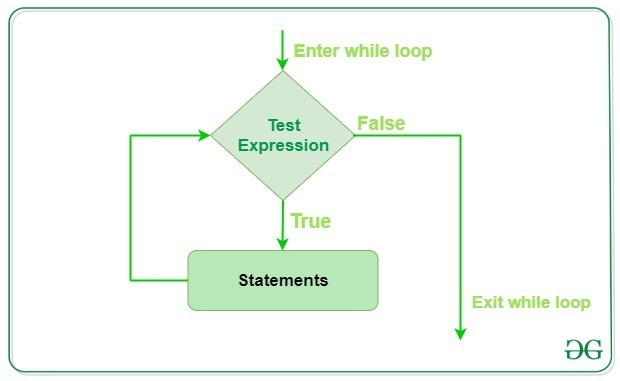```
# Python program to illustrate
# while and while-else loop
i = 0
while (i < 3):
i = i + 1
print("Hello Geek")

# checks if list still
# contains any element
a = [1, 2, 3, 4]
while a:
print(a.pop())

i = 10
while i < 12:
i += 1
print(i)
break
else: # Not executed as there is a break
print("No Break")

```

Output:

```Hello Geek
Hello Geek
Hello Geek
4
3
2
1
11
```

• For and for-else loop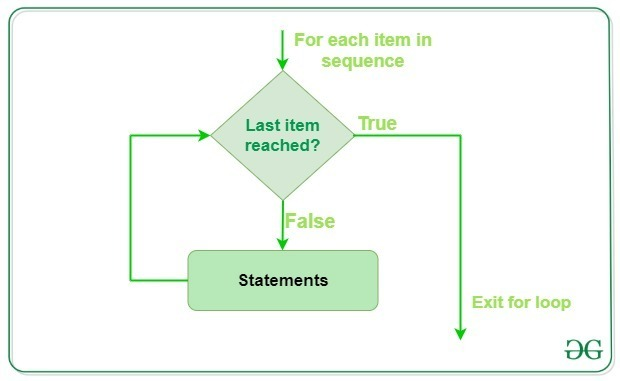```
# Python program to illustrate
# Iterating over a list
print("List Iteration")
l = ["geeks", "for", "geeks"]
for i in l:
print(i)

# Iterating over a String
print("\nString Iteration")
s = "Geeks"
for i in s :
print(i)

print("\nFor-else loop")
for i in s:
print(i)
else: # Executed because no break in for
print("No Break\n")

for i in s:
print(i)
break
else: # Not executed as there is a break
print("No Break")

```

Output:

```List Iteration
geeks
for
geeks

String Iteration
G
e
e
k
s

For-else loop
G
e
e
k
s
No Break

G
```

• range() function: `range()` allows user to generate a series of numbers within a given range. Depending on how many arguments user is passing to the function. This function takes three arguments.1) start: integer starting from which the sequence of integers is to be returned
2) stop: integer before which the sequence of integers is to be returned.
3) step: integer value which determines the increment between each integer in the sequence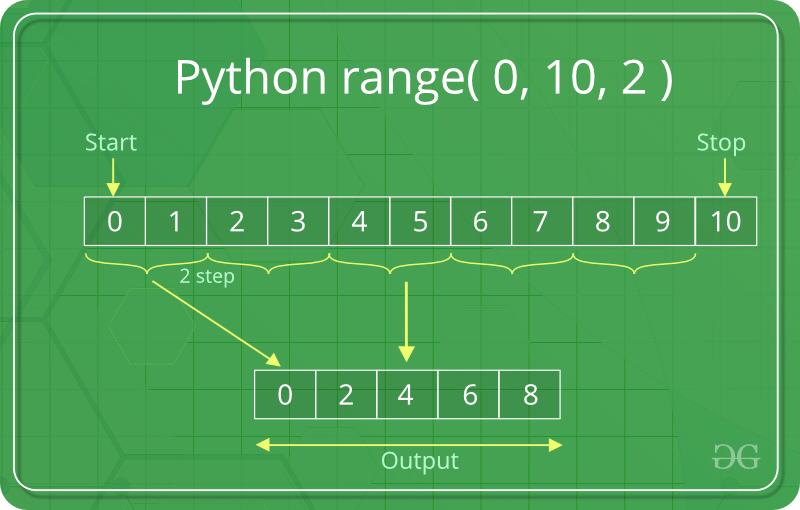```
# Python program to demonstrate
# range() function

for i in range(5):
print(i, end =" ")
print()

for i in range(2, 9):
print(i, end =" ")
print()

# incremented by 3
for i in range(15, 25, 3):
print(i, end =" ")

```

Output:

```0 1 2 3 4
2 3 4 5 6 7 8
15 18 21 24
```

Refer to the below articles to know more about Loops:

## Loop control statements

Loop control statements change execution from its normal sequence. Following are the loop control statements provided by Python:

• Break: Break statement in Python is used to bring the control out of the loop when some external condition is triggered.
• Continue: Continue statement is opposite to that of break statement, instead of terminating the loop, it forces to execute the next iteration of the loop.
• Pass: Pass statement is used to write empty loops. Pass is also used for empty control statement, function and classes.
```
# Python program to demonstrate
# break, continue and pass

s = 'geeksforgeeks'

for letter in s:
if letter == 'e' or letter == 's':
break
print(letter, end = " ")
print()

for letter in s:
if letter == 'e' or letter == 's':
continue
print(letter, end = " ")
print()

for letter in s:
if letter == 'e' or letter == 's':
pass
print(letter, end = " ")

```

Output:

```g
g k f o r g k
g e e k s f o r g e e k s
```

Note: For more information, refer 👉🏽 break, continue and pass in Python.

# Functions

Functions are generally the block of codes or statements in a program that gives the user the ability to reuse the same code which ultimately saves the excessive use of memory, acts as a time saver and more importantly, provides better readability of the code. So basically, a function is a collection of statements that perform some specific task and return the result to the caller. A function can also perform some specific task without returning anything. In Python, `def` keyword is used to create functions.

```
# Python program to demonstrate
# functions

# Defining functions
print("Hello Geeks")

# Function that returns sum
# of first 10 numbers
def my_func():
a = 0
for i in range(1, 11):
a = a + i
return a

# Calling functions
res = my_func()
print(res)

```

Output:

```Hello Geeks
55
```

## Function with arguments

• Default arguments: A default argument is a parameter that assumes a default value if a value is not provided in the function call for that argument.
```
# Python program to demonstrate
# default arguments

def myFun(x, y = 50):
print("x: ", x)
print("y: ", y)

# Driver code
myFun(10)

```

Output:

```('x: ', 10)
('y: ', 50)
```
• Keyword arguments: The idea is to allow caller to specify argument name with values so that caller does not need to remember order of parameters.
```
# Python program to demonstrate Keyword Arguments
def student(firstname, lastname):
print(firstname, lastname)

# Keyword arguments
student(firstname ='Geeks', lastname ='Practice')
student(lastname ='Practice', firstname ='Geeks')

```

Output:

```('Geeks', 'Practice')
('Geeks', 'Practice')
```
• Variable length arguments: In Python a function can also have variable number of arguments. This can be used in the case when we do not know in advance the number of arguments that will be passed into a function.
```
# Python program to demonstrate
# variable length arguments

# variable arguments
def myFun1(*argv):
for arg in argv:
print(arg, end =" ")

# variable keyword arguments
def myFun2(**kwargs):
for key, value in kwargs.items():
print ("% s == % s" %(key, value))

# Driver code
myFun1('Hello', 'Welcome', 'to', 'GeeksforGeeks')
print()
myFun2(first ='Geeks', mid ='for', last ='Geeks')

```

Output:

```Hello Welcome to GeeksforGeeks
first == Geeks
last == Geeks
mid == for
```

Refer to the below articles to know more about functions:

## Lambda functions

In Python, the lambda/anonymous function means that a function is without a name. The `lambda` keyword is used to create anonymous functions. Lambda function can have any number of arguments but has only one expression.

```
# Python code to demonstrate
# labmda function

# Cube using lambda
cube = lambda x: x * x*x
print(cube(7))

# List comprehension using lambda
a = [(lambda x: x * 2)(x) for x in range(5)]
print(a)

```

Output:

```343
[0, 2, 4, 6, 8]
```

Refer to the below articles to know more about Lambda:

# Object Oriented Programming

Object-oriented programming aims to implement real-world entities like inheritance, hiding, polymorphism, etc in programming. The main aim of OOP is to bind together the data and the functions that operate on them so that no other part of the code can access this data except that function.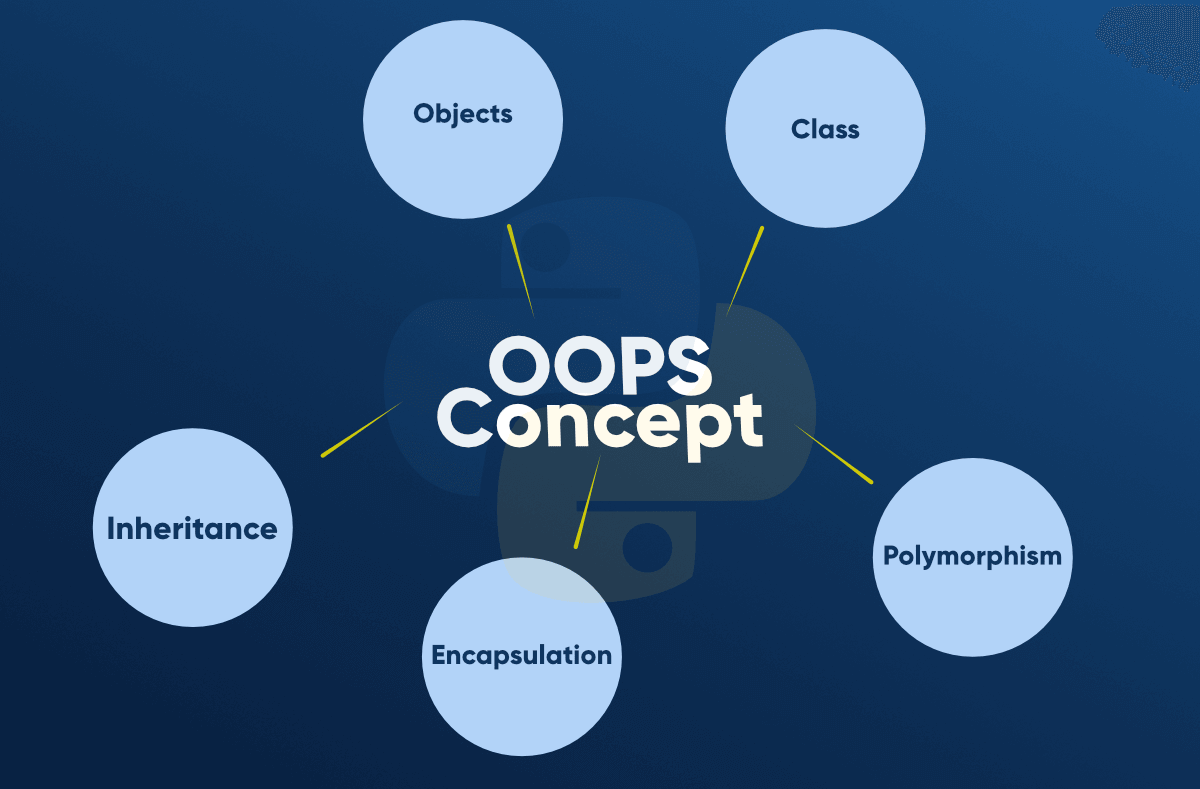## Classes and Objects

Class creates a user-defined data structure, which holds its own data members and member functions, which can be accessed and used by creating an instance of that class. A class is like a blueprint for an object.

An Object is an instance of a Class. A class is like a blueprint while an instance is a copy of the class with actual values.

```
# Python program to demonstrate
# classes and objects

class Dog:

# A simple class attribute
attr1 = "mamal"
attr2 = "dog"

# A sample method
def fun(self):
print("I'm a", self.attr1)
print("I'm a", self.attr2)

# Driver code
# Object instantiation
Rodger = Dog()

# Accessing class attributes
# and method through objects
print(Rodger.attr1)
Rodger.fun()

```

Output:

```mamal
I'm a mamal
I'm a dog
```

The self

self represents the instance of the class. By using the “`self`” keyword we can access the attributes and methods of the class in python. It binds the attributes with the given arguments.

## Constructors and Destructors

Constructors: Constructors are generally used for instantiating an object.The task of constructors is to initialize(assign values) to the data members of the class when an object of class is created. In Python the `__init__()` method is called the constructor and is always called when an object is created. There can be two types of constructors:

• Default constructor: The constructor which is called implicilty and do not accept any argument.
• Parameterized constructor:Constructor which is called explicitly with parameters is known as parameterized constructor.
```# Python program to demonstrate
# constructors

# parameterized constructor
def __init__(self, f, s):
self.first = f
self.second = s

def calculate(self):
print(self.first + self.second)

# Invoking parameterized constructor

obj.calculate()
```

Output:

```3000
```

Destructors: Destructors are called when an object gets destroyed. The `__del__()` method is a known as a destructor method in Python. It is called when all references to the object have been deleted i.e when an object is garbage collected.

```
# Python program to illustrate destructor
class Employee:

# Initializing
def __init__(self):
print('Employee created.')

# Deleting (Calling destructor)
def __del__(self):
print('Destructor called, Employee deleted.')

obj = Employee()
del obj

```

Output:

```Employee created.
Destructor called, Employee deleted.
```

## Inheritance

Inheritance is the ability of any class to extract and use features of other classes. It is the process by which new classes called the derived classes are created from existing classes called Base classes.

```
# A Python program to demonstrate inheritance

class Person():

# Constructor
def __init__(self, name):
self.name = name

# To get name
def getName(self):
return self.name

# To check if this person is employee
def isEmployee(self):
return False

# Inherited or Sub class (Note Person in bracket)
class Employee(Person):

# Here we return true
def isEmployee(self):
return True

# Driver code
emp = Person("Geek1")  # An Object of Person
print(emp.getName(), emp.isEmployee())

emp = Employee("Geek2") # An Object of Employee
print(emp.getName(), emp.isEmployee())

```

Output:

```Geek1 False
Geek2 True
```

## Encapsulation

Encapsulation describes the idea of wrapping data and the methods that work on data within one unit. This puts restrictions on accessing variables and methods directly and can prevent the accidental modification of data.

```
# Python program to demonstrate
# encapsulation

# Creating a Base class
class Base:
def __init__(self):
self.a = "GeeksforGeeks"
self.__c = "GeeksforGeeks"

# Creating a derived class
class Derived(Base):
def __init__(self):

# Calling constructor of
# Base class
Base.__init__(self)
print("Calling private member of base class: ")
print(self.__a)
# Driver code
obj = Derived()

```

Output:

```Traceback (most recent call last):
File "/home/5a605c59b5b88751d2b93dd5f932dbd5.py", line 20, in
obj = Derived()
File "/home/5a605c59b5b88751d2b93dd5f932dbd5.py", line 18, in __init__
print(self.__a)
AttributeError: 'Derived' object has no attribute '_Derived__a'
```

## Polymorphism

Polymorphism refers to the ability of OOPs programming languages to differentiate between entities with the same name efficiently. This is done by Python with the help of the signature of these entities.

```
# Python program to demonstrate
# Polymorphism

class A():
def show(self):
print("Inside A")

class B():
def show(self):
print("Inside B")

# Driver's code
a = A()
a.show()
b = B()
b.show()

```

Output:

```Inside A
Inside B
```

Refer to the articles to know more about OOPS:

# File Handling

File handling is the ability of Python to handle files i.e. to read and write files along with many other file handling options. Python treats files differently as text or binary and this is important. Each line of code includes a sequence of characters and they form a text file. Each line of a file is terminated with a special character, called the EOL or End of Line characters like comma `{, }` or newline character.

Basic File Handling operations in Python are:

1) Open a file: Opening a file refers to getting the file ready either for reading or for writing. This can be done using the `open()` function. This function returns a file object and takes two arguments, one that accepts the file name and another that accepts the mode(Access Mode). Python provides six Access Modes:

Access Mode Description
Read Only (‘r’) Open text file for reading. The handle is positioned at the beginning of the file.
Read and Write (‘r+’) Open the file for reading and writing. The handle is positioned at the beginning of the file.
Write Only (‘w’) Open the file for writing. For existing file, the data is truncated and over-written. The handle is positioned at the beginning of the file.
Write and Read (‘w+’) Open the file for reading and writing. For existing file, data is truncated and over-written. The handle is positioned at the beginning of the file.
Append Only (‘a’) Open the file for writing. The handle is positioned at the end of the file.
Append and Read (‘a+’) Open the file for reading and writing. The handle is positioned at the end of the file.
```
# Open function to open the file "MyFile1.txt"
# (same directory) in read mode and
file1 = open("MyFile.txt", "r")

# store its reference in the variable file1
# and "MyFile2.txt" in D:\Text in file2
file2 = open(r"D:\Text\MyFile2.txt", "r+")
```

2) Close the file: `close()` function closes the file and frees the memory space acquired by that file.

```
# Opening and Closing a file "MyFile.txt"
# for object name file1.
file1 = open("MyFile.txt", "a")
file1.close()

```

3) Reading from a File: There are three ways to read data from a text file.

`File_object.read([n])`
• readline(): Reads a line of the file and returns in form of a string.For specified n, reads at most n bytes. However, does not reads more than one line, even if n exceeds the length of the line.
`File_object.readline([n])`
• readlines(): Reads all the lines and return them as each line a string element in a list.
`File_object.readlines()`

Let’s suppose the file looks like this: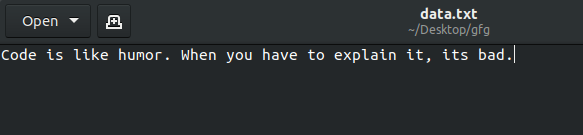```
# Program to show various ways to
# read data from a file.

file1 = open("data.txt", "r+")

print("Output of Read function is ")
print()

# seek(n) takes the file handle to the nth
# bite from the beginning.
file1.seek(0)

print("Output of Readline function is ")
print()

file1.seek(0)

print("Output of Readlines function is ")
print()
file1.close()

```

Output:

```Output of Read function is
Code is like humor. When you have to explain it, its bad.

Code is like humor. When you have to explain it, its bad.

['Code is like humor. When you have to explain it, its bad.']
```

4) Writing to a file: There are two ways to write in a file.

• write(): Inserts the string str1 in a single line in the text file.
`File_object.write(str1)`
• writelines(): For a list of string elements, each string is inserted in the text file. Used to insert multiple strings at a single time.
`File_object.writelines(L) for L = [str1, str2, str3]`
```
# Python program to demonstrate
# writing to file

# Opening a file
file1 = open('myfile.txt', 'w')
L = ["This is Delhi \n", "This is Paris \n", "This is London \n"]
s = "Hello\n"

# Writing a string to file
file1.write(s)

# Writing multiple strings
# at a time
file1.writelines(L)

# Closing file
file1.close()

```

Output: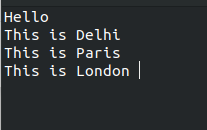Refer to the below articles to know more about File-Handling:

# Modules and Packages

## Modules

A module is a self-contained Python file that contains Python statements and definitions, like a file named `GFG.py`, which can be considered as a module named `GFG` which can be imported with the help of `import statement`.

Let’s create a simple module named GFG.

```
# Python program to demonstrate
# modules

# Defining a function
def Geeks():
print("GeeksforGeeks")

# Defining a variable
location = "Noida"

# Defining a class
class Employee():

def __init__(self, name, position):
self. name = name
self.position = position

def show(self):
print("Employee name:", self.name)
print("Employee position:", self.position)
```

To use the above created module, create a new Python file in the same directory and import GFG module using the `import` statement.

```
# Python program to demonstrate
# modules

import GFG

# Use the function created
GFG.Geeks()

# Print the variable declared
print(GFG.location)

# Use the class created
emp = GFG.Employee("Nikhil", "Developer")
emp.show()

```

Output:

```GeeksforGeeks
Noida
Employee name: Nikhil
Employee position: Developer
```

## Packages

Packages are a way of structuring many packages and modules which helps in a well-organized hierarchy of data set, making the directories and modules easy to access.

To create a package in Python, we need to follow these three simple steps:

• First, we create a directory and give it a package name, preferably related to its operation.
• Then we put the classes and the required functions in it.
• Finally we create an `__init__.py` file inside the directory, to let Python know that the directory is a package.

Example: Let’s create a package for cars.

• First we create a directory and name it Cars.
• Then we need to create modules. We will create 2 modules – BMW and AUDI.For Bmw.py
```
# Python code to illustrate the Modules
class Bmw:

def __init__(self):
self.models = ['i8', 'x1', 'x5', 'x6']

def outModels(self):
print('These are the available models for BMW')
for model in self.models:
print('\t % s ' % model)

```

For Audi.py

```
# Python code to illustrate the Module
class Audi:

def __init__(self):
self.models = ['q7', 'a6', 'a8', 'a3']

def outModels(self):
print('These are the available models for Audi')
for model in self.models:
print('\t % s ' % model)

```
• Finally we create the __init__.py file. This file will be placed inside the Cars directory and can be left blank.

Now, let’s use the package that we created. To do this make a sample.py file in the same directory where Cars package is located and add the following code to it:

```
# Import classes from your brand new package
from Cars import Bmw
from Cars import Audi

# Create an object of Bmw class & call its method
ModBMW = Bmw()
ModBMW.outModels()

# Create an object of Audi class & call its method
ModAudi = Audi()
ModAudi.outModels()

```

Output: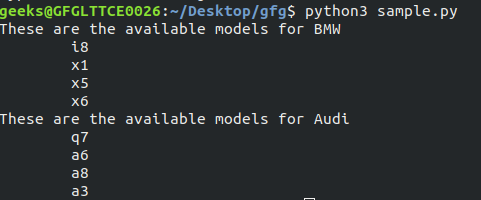Note: For more information, refer 👉🏽 Create and Access a Python Package.

# Regular expressions

Module Regular Expressions(RE) specifies a set of strings(pattern) that matches it. To understand the RE analogy, MetaCharacters are useful, important and will be used in functions of module `re`. There are a total of 14 metacharacters:

```\   Used to drop the special meaning of character
following it (discussed below)
[]  Represent a character class
^   Matches the beginning
\$   Matches the end
.   Matches any character except newline
?   Matches zero or one occurrence.
|   Means OR (Matches with any of the characters
separated by it.
*   Any number of occurrences (including 0 occurrences)
+   One ore more occurrences
{}  Indicate the number of occurrences of a preceding RE
to match.
()  Enclose a group of REs
```

The most frequently used methods are:

• re.findall(): Return all non-overlapping matches of pattern in string, as a list of strings. The string is scanned left-to-right, and matches are returned in the order found.
```
# A Python program to demonstrate working of
# findall()
import re

string = """Hello my Number is 123456789 and
my friend's number is 987654321"""

# A sample regular expression to find digits.
regex = '\d+'

match = re.findall(regex, string)
print(match)

```

Output:

```['123456789', '987654321']
```

In the above example, metacharacter blackslash `‘\’` has a very important role as it signals various sequences. If the blackslash is to be used without its special meaning as metacharacter, use`’\\’`.

```\d   Matches any decimal digit, this is equivalent
to the set class [0-9].
\D   Matches any non-digit character.
\s   Matches any whitespace character.
\S   Matches any non-whitespace character
\w   Matches any alphanumeric character, this is
equivalent to the class [a-zA-Z0-9_].
\W   Matches any non-alphanumeric character.
```
• re.compile(): Regular expressions are compiled into pattern objects, which have methods for various operations such as searching for pattern matches or performing string substitutions.
```
# A Python program to demonstrate working of
# compile()
import re

# it is equivalent to [abcde].
p = re.compile('[a-e]')

print(p.findall("Aye, said Mr. Gibenson Stark"))

```

Output:

```['e', 'a', 'd', 'b', 'e', 'a']
```
• re.match(): This function attempts to match pattern to whole string. The re.match function returns a match object on success, None on failure.
```
# A Python program to demonstrate working
# of re.match().
import re

def findMonthAndDate(string):

regex = r"([a-zA-Z]+) (\d+)"
match = re.match(regex, string)

if match == None:
print("Not a valid date")
return

print("Given Data: % s" % (match.group()))
print("Month: % s" % (match.group(1)))
print("Day: % s" % (match.group(2)))

# Driver Code
findMonthAndDate("Jun 24")
print("")
findMonthAndDate("I was born on June 24")

```

Output:

```Given Data: Jun 24
Month: Jun
Day: 24

Not a valid date
```
• re.search(): This method either returns None (if the pattern doesn’t match), or a re.MatchObject that contains information about the matching part of the string.
```
# A Python program to demonstrate working of re.match().
import re

regex = r"([a-zA-Z]+) (\d+)"

match = re.search(regex, "I was born on June 24")

if match != None:

print("Match at index % s, % s" % (match.start(), match.end()))

# this will print "June 24"
print("Full match: % s" % (match.group(0)))

# this will print "June"
print("Month: % s" % (match.group(1)))

# this will print "24"
print("Day: % s" % (match.group(2)))

else:
print("The regex pattern does not match.")

```

Output:

```Match at index 14, 21
Full match: June 24
Month: June
Day: 24
```

# Exception handling

Like other languages, Python also provides the runtime errors via exception handling method with the help of try-except.

How try-except works?

• First try clause is executed i.e. the code between try and except clause.
• If there is no exception, then only try clause will run, except clause is finished.
• If any exception occurred, try clause will be skipped and except clause will run.
• If any exception occurs, but the except clause within the code doesn’t handle it, it is passed on to the outer try statements. If the exception left unhandled, then the execution stops.
• A try statement can have more than one except clause.

Code 1: No exception, so try clause will run.

```
# Python code to illustrate
# working of try()
def divide(x, y):
try:
result = x // y
except ZeroDivisionError:
print("Sorry ! You are dividing by zero ")

# Look at parameters and note the working of Program
divide(3, 2)

```

Output:

```Yeah ! Your answer is : 1
```

Code 2: There is an exception so only except clause will run.

```
# Python code to illustrate
# working of try()
def divide(x, y):
try:
result = x // y
except:
print("Sorry ! You are dividing by zero ")

# Look at parameters and note the working of Program
divide(3, 0)

```

Output:

```Sorry ! You are dividing by zero
```

Else Clause: In python, you can also use else clause on try-except block which must be present after all the except clauses. The code enters the else block only if the try clause does not raise an exception.

```
# Python code to illustrate
# working of try()
def divide(x, y):
try:
result = x // y
except:
print("Sorry ! You are dividing by zero ")
else:
print("No exception raised")

# Look at parameters and note the working of Program
divide(3, 2)

```

Output:

```Yeah ! Your answer is : 1
No exception raised
```

Raising Exception: The raise statement allows the programmer to force a specific exception to occur. This must be either an exception instance or an exception class. To know more about the list of exception class 👉🏽 click here.

```
# Program to depict Raising Exception

try:
raise NameError("Hi there")  # Raise Error
except NameError:
print("An exception")
raise  # To determine whether the exception was raised or not

```

Output:

```Traceback (most recent call last):
File "/home/4678cd3d633b2ddf9d19fde6283f987b.py", line 4, in
raise NameError("Hi there")  # Raise Error
NameError: Hi there
```